# Test: Measurement of Power & Energy

## 10 Questions MCQ Test Topicwise Question Bank for Electronics Engineering | Test: Measurement of Power & Energy

Description
Attempt Test: Measurement of Power & Energy | 10 questions in 30 minutes | Mock test for Electrical Engineering (EE) preparation | Free important questions MCQ to study Topicwise Question Bank for Electronics Engineering for Electrical Engineering (EE) Exam | Download free PDF with solutions
QUESTION: 1

### Which one of the following meters is an induction type instrument?

Solution:

An energymeter is an induction and an integrating type instrument.

QUESTION: 2

### The error caused by pressure coil inductance in

Solution:

To reduce the error in the wattmeter reading due to pressure coil inductance, the wattmeters are compensated by connecting a capacitor in parallel with a portion of series resistance (multiplier) as shown below.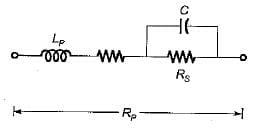QUESTION: 3

### Power at RF is measured using

Solution:

At AF (audiofrequency) and RF (radio frequency) it is easier to measure voltage, current and impedance, than to measure- power. Direct measurement of power is not done in this range. Here, power is calculated from the equation
P = E2IR or P = I2 R

QUESTION: 4

Creeping in a single phase induction type energy meter may be due to

Solution:
QUESTION: 5

In a single phase induction type energy meter, the lag adjustment is done

Solution:

For the energy meter to record true energy the pressure coil should be highly inductive (i.e, Δ = 90°). Thus, for the angle between the shunt magnet flux (φρ) and the supply voltage to be 90°, a lagging coil is placed between the potential coil and the rotating aluminium disc. Lag adjustment sometimes is called power factor or quadrature or inductive load adjustment.

QUESTION: 6

A wattmeter has a current coil of 0.1 Ω resistance and a pressure coil of 6500 Ω respectively. The wattmeter shows a reading of 12 A at 250 V with unity power factor. The percentage errors, due to resistance only with each of the two methods of connections shown below will be respectively

Solution: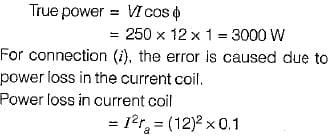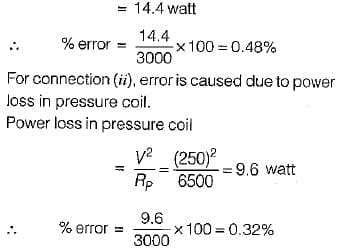QUESTION: 7

The power factor in a 3-phase circuit using two wattmeter method is given by

Solution:

From two-wattmeter method of measurement of power, we have: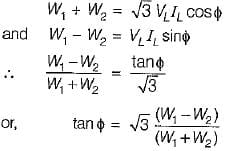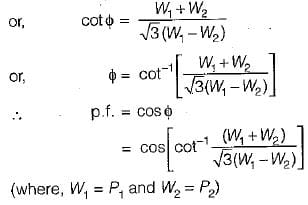QUESTION: 8

Phantom leading for testing of energy meter is used

Solution:

When the energy meter is tested under high current rating, there is lot of waste of power. To reduce this unwanted power loss, Phantom or Fictitious loading is done.

QUESTION: 9

The braking torque provided by a permanent magnet in a single phase meter is proportional to the

Solution:

Braking torque provided by the permanent magnet is given by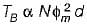Where,
N = speed of the disc in rpm
φm = maximum value of flux of the permanent magnet
d = distance of the permanent magnet from the centre of the revolving disc

QUESTION: 10

The meter constant of a 230 V, 10 A watthour meter is 1800 revolutions per kWh. The meter is tested at half load and rated voltage and unity power factor. The meter is found to make 80 rev. in 138 seconds. The meter error at half load is

Solution:

Given, energy meter constant,
K = 1800 revolutions per kWh Actuai energy or true energy consumed by the energy meter at half load during 138 seconds is: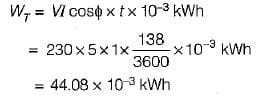Also, measured energy or energy recorded is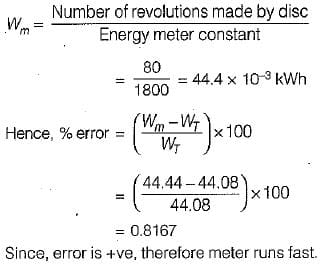Use Code STAYHOME200 and get INR 200 additional OFF Use Coupon Code